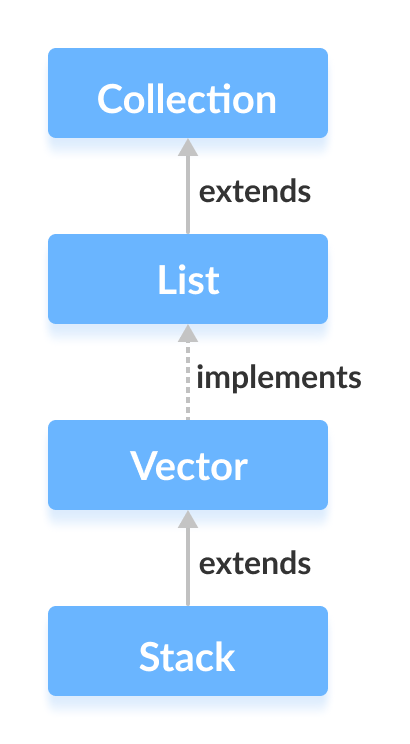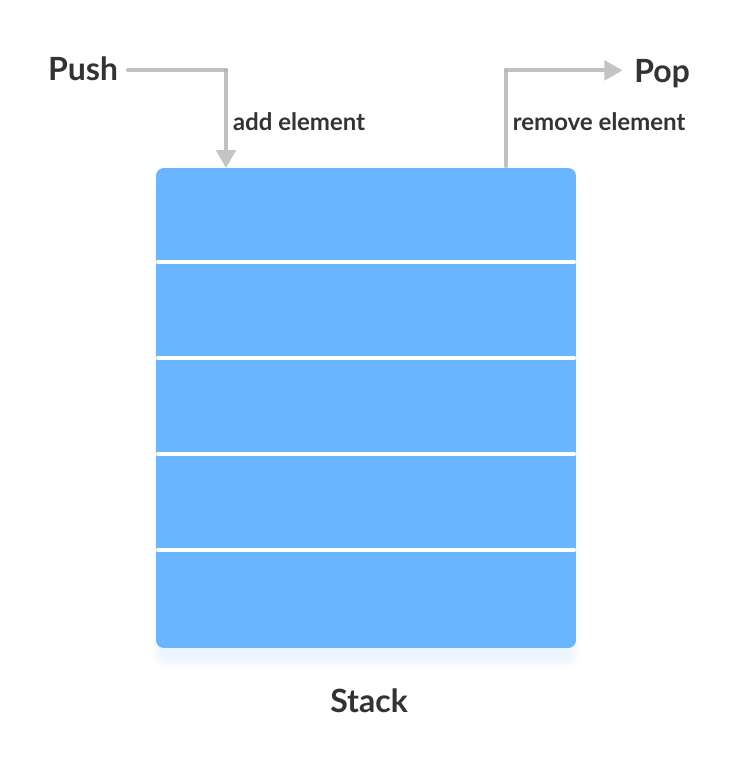# Java Stack Class

In this tutorial, we will learn about the Java Stack class and its methods with the help of examples.

The Java collections framework has a class named `Stack` that provides the functionality of the stack data structure.

The `Stack` class extends the `Vector` class.## Stack Implementation

In stack, elements are stored and accessed in Last In First Out manner. That is, elements are added to the top of the stack and removed from the top of the stack.## Creating a Stack

In order to create a stack, we must import the `java.util.Stack` package first. Once we import the package, here is how we can create a stack in Java.

``````Stack<Type> stacks = new Stack<>();
``````

Here, `Type` indicates the stack's type. For example,

``````// Create Integer type stack
Stack<Integer> stacks = new Stack<>();

// Create String type stack
Stack<String> stacks = new Stack<>();
``````

## Stack Methods

Since `Stack` extends the `Vector` class, it inherits all the methods `Vector`. To learn about different `Vector` methods, visit Java Vector Class.

Besides these methods, the `Stack` class includes 5 more methods that distinguish it from `Vector`.

### push() Method

To add an element to the top of the stack, we use the `push()` method. For example,

``````import java.util.Stack;

class Main {
public static void main(String[] args) {
Stack<String> animals= new Stack<>();

animals.push("Dog");
animals.push("Horse");
animals.push("Cat");

System.out.println("Stack: " + animals);
}
}
``````

Output

```Stack: [Dog, Horse, Cat]
```

### pop() Method

To remove an element from the top of the stack, we use the `pop()` method. For example,

``````import java.util.Stack;

class Main {
public static void main(String[] args) {
Stack<String> animals= new Stack<>();

animals.push("Dog");
animals.push("Horse");
animals.push("Cat");
System.out.println("Initial Stack: " + animals);

// Remove element stacks
String element = animals.pop();
System.out.println("Removed Element: " + element);
}
}
``````

Output

```Initial Stack: [Dog, Horse, Cat]
Removed Element: Cat
```

### peek() Method

The `peek()` method returns an object from the top of the stack. For example,

``````import java.util.Stack;

class Main {
public static void main(String[] args) {
Stack<String> animals= new Stack<>();

animals.push("Dog");
animals.push("Horse");
animals.push("Cat");
System.out.println("Stack: " + animals);

// Access element from the top
String element = animals.peek();
System.out.println("Element at top: " + element);

}
}
``````

Output

```Stack: [Dog, Horse, Cat]
Element at top: Cat
```

To search an element in the stack, we use the `search()` method. It returns the position of the element from the top of the stack. For example,

``````import java.util.Stack;

class Main {
public static void main(String[] args) {
Stack<String> animals= new Stack<>();

animals.push("Dog");
animals.push("Horse");
animals.push("Cat");
System.out.println("Stack: " + animals);

// Search an element
int position = animals.search("Horse");
System.out.println("Position of Horse: " + position);
}
}
``````

Output

```Stack: [Dog, Horse, Cat]
Position of Horse: 2
```

### empty() Method

To check whether a stack is empty or not, we use the `empty()` method. For example,

``````import java.util.Stack;

class Main {
public static void main(String[] args) {
Stack<String> animals= new Stack<>();

animals.push("Dog");
animals.push("Horse");
animals.push("Cat");
System.out.println("Stack: " + animals);

// Check if stack is empty
boolean result = animals.empty();
System.out.println("Is the stack empty? " + result);
}
}
``````

Output

```Stack: [Dog, Horse, Cat]
Is the stack empty? false
```

## Use ArrayDeque Instead of Stack

The `Stack` class provides the direct implementation of the stack data structure. However, it is recommended not to use it. Instead, use the `ArrayDeque` class (implements the `Deque` interface) to implement the stack data structure in Java.